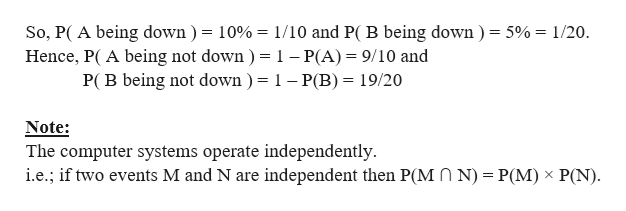A firm has two computer systems available for processing telephone orders. At any given time, system A has a 10% chance of being “down”, while system B has just a 5% chance of being “down”. The computer systems operate independently. For a typical telephone order, determine the following:What is the probability that exactly one of the computer systems will be operational

Question

A firm has two computer systems available for processing telephone orders. At any given time, system A has a 10% chance of being “down”, while system B has just a 5% chance of being “down”. The computer systems operate independently. For a typical telephone order, determine the following:

What is the probability that exactly one of the computer systems will be operational

Step 1

We are given that a firm has two computer systems available for processing telephone orders. At any given time, syste...help_outlineImage TranscriptioncloseSo, P( A being down ) = 10%= 1/10 and P( B being down) = 5%= 1/20 Hence, P( A being not down ) = 1 - P(A) 9/10 and P( B being not down ) = 1 - P(B)= 19/20 Note: The computer systems operate independently i.e.; if two events M and N are independent then P(MO N) = P(M) x P(N) fullscreen

Want to see the full answer?

See Solution

Want to see this answer and more?

Our solutions are written by experts, many with advanced degrees, and available 24/7

See Solution
Tagged in

Basic Probability